E8 Next: Conclusions Up: Exceptional Lie Algebras Previous: E7

## 4.6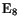With 248 dimensions,is the biggest of the exceptional Lie groups, and in some ways the most mysterious. The easiest way to understand a group is to realize it as as symmetries of a structure one already understands. Of all the simple Lie groups,is the only one whose smallest nontrivial representation is the adjoint representation. This means that in the context of linear algebra,is most simply described as the group of symmetries of its own Lie algebra! One way out of this vicious circle would be to describeas isometries of a Riemannian manifold. As already mentioned,is the isometry group of a 128-dimensional manifold called. But alas, nobody seems to know how to definewithout first defining. Thus this group remains a bit enigmatic.

At present, to get our hands onwe must start with its Lie algebra. We can define this using any of the three equivalent magic square constructions explained in the previous section. Vinberg's construction gives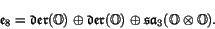Tits' construction gives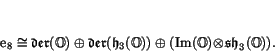The Barton-Sudbery construction gives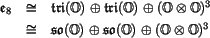We can use any of these to count the dimension of; for example, the last one gives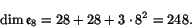To emphasize the importance of triality, we can rewrite the the Barton-Sudbery description ofas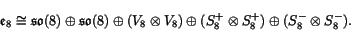Here the Lie bracket is built from natural maps relating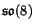and its three 8-dimensional irreducible representations. In particular,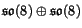is a Lie subalgebra, and the first copy ofacts on the first factor in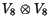,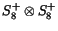, and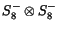, while the second copy acts on the second factor in each of these. This has a pleasant resemblance to the triality description of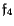that we found in Section 4.2:Now let us turn from 8-dimensional geometry to 16-dimensional geometry. On the one hand, we haveOn the other hand, we have seen thatComparing these, it is natural to hope thatcontainsas a Lie subalgebra. In fact this is true! Even better, if we restrict the right-handed spinor representation ofto, it decomposes as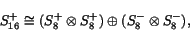so we obtain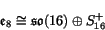or in more octonionic language,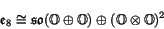where we use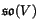to mean the Lie algebra of skew-adjoint real-linear transformations of the real inner product space.

The really remarkable thing about the isomorphismis that the Lie bracket inis entirely built from natural maps involvingand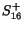: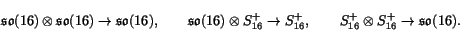The first of these is the Lie bracket in, the second is the action ofon its right-handed spinor representation, and the third is obtained from the second by duality, using the natural inner product onandto identify these spaces with their duals. In fact, this is a very efficient way to define. If we take this approach, we must verify the Jacobi identity: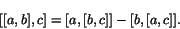When all three of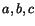lie inthis is just the Jacobi identity for. When two of them lie in, it boils down to fact that spinors indeed form a representation of. Thanks to duality, the same is true when just one lies in. It thus suffices to consider the case whenall lie in. This is the only case that uses anything special about the number 16. Unfortunately, at this point a brute-force calculation seems to be required. For two approaches that minimize the pain, see the books by Adams  and by Green, Schwarz and Witten . It would be nice to find a more conceptual approach.

Starting from, we can defineto be the simply-connected Lie group with this Lie algebra. As shown by Adams , the subgroup ofgenerated by the Lie subalgebra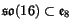is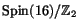. This lets us define the octooctonionic projective plane by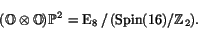The tangent space at any point of this manifold is isomorphic to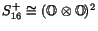. This partially justifies calling it 'octooctonionic projective plane', though it seems not to satisfy the usual axioms for a projective plane.

We can put an-invariant Riemannian metric on the octooctonionic projective plane by the technique of averaging over the group action. It then turns out  that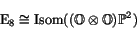and thusSummarizing, we have the following octonionic descriptions of:

Theorem 8.   The compact real form ofis given bywhere in each case the Lie bracket onis built from natural bilinear operations on the summands.

Next: Conclusions Up: Exceptional Lie Algebras Previous: E7

© 2001 John Baez